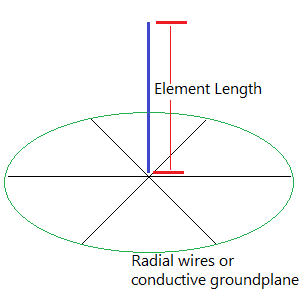# Quarter-Wave Vertical Antenna Length Calculator

A quarter-wavelength antenna is easy and inexpensive to build and can be an effective antenna with a low angle of radiation (that is, power is radiated out, not up).

The formula for calculating the approximate length of a quarter-wave vertical antenna is:

Quarter-wavelength in feet: 234 / frequency in MHz

Quater-wavelength in meters: 71.5 / frequency in MHzTo use the calculator, enter the desired operating frequency in megahertz to get a starting length in both feet and meters for building a quarter-wave vertical antenna .

Frequency in MHz:

Length of the vertical element:
-
-

You can shorten your vertical antenna by using a loading coil. Use the Coil-Shortened Vertical Antenna Calculator to see how.

The calculated length is approximate. In practice it's best to make the antenna a little longer than the calculated value and then trim it to get the best SWR value.

Note that the quality of "the other half" of the antenna--the conductive ground plane beneath it--makes a big difference in both the efficiency and feedpoint impedance of the antenna. The ideal groundplane would be a conductive sheet. The more radial wires that you can lay down to approximate that ideal groundplane, the more efficient your antenna will be. Radial wires should ideally be at least one quarter-wavelength long (that is, the same length as the antenna or longer).

Raising the antenna about ground, with the radial wires descending at an angle, also improves efficency.

Increasing the size of the conductor will increase the usable frequency range of the antenna (the bandwidth), so at frequencies of about 10 megahertz or higher, consider using copper or aluminum tubing instead of wire.

Reference: The ARRL Antenna Book for Radio Communications